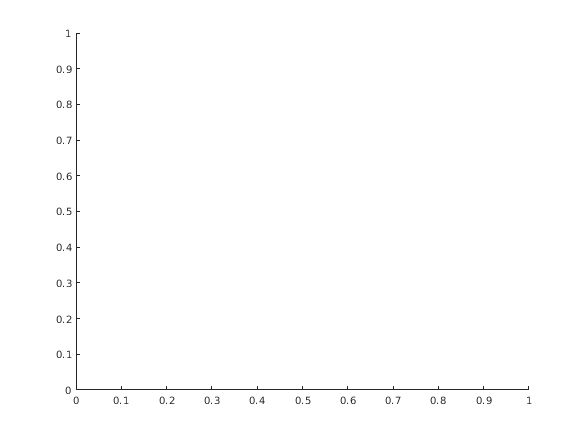Cody

# Problem 1503. Perl 2: pop

Solution 854642

Submitted on 22 Mar 2016 by LY Cao
• Size: 26
• This is the leading solution.
This solution is locked. To view this solution, you need to provide a solution of the same size or smaller.

### Test Suite

Test Status Code Input and Output
1   Pass
x=[1 2 3 4 5] [a, x]=pop(x); a_correct = 5; x_correct = [1 2 3 4]; assert(isequal(a, a_correct)); assert(isequal(x, x_correct));

x = 1 2 3 4 5

``
2   Pass
x=[] [a,x]=pop(x); a_correct = []; x_correct = []; assert(isequal(a, a_correct)); assert(isequal(x, x_correct));

x = []

3   Pass
x= [a,x]=pop(x); a_correct = 1; x_correct = []; assert(isequal(a, a_correct)); assert(isequal(x, x_correct));

x = 1

### Community Treasure Hunt

Find the treasures in MATLAB Central and discover how the community can help you!

Start Hunting!